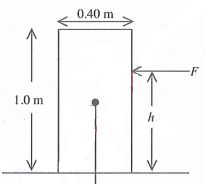Problem: In the figure, a uniform rectangular crate 0.40 m wide and 1.0 m tall rests on a horizontal surface. The crate weighs 930 N, and its center of gravity is at its geometric center. A horizontal force F is applied at a distance h above the floor. If h = 0.61 m, what minimum value of F is required to make the crate start to tip over? Static friction is large enough that the crate does not start to slide. A) 763 N B) 688 N C) 305 N D) 413 N

82% (32 ratings)
Problem Details

In the figure, a uniform rectangular crate 0.40 m wide and 1.0 m tall rests on a horizontal surface. The crate weighs 930 N, and its center of gravity is at its geometric center. A horizontal force F is applied at a distance h above the floor. If h = 0.61 m, what minimum value of F is required to make the crate start to tip over? Static friction is large enough that the crate does not start to slide.

A) 763 N

B) 688 N

C) 305 N

D) 413 N